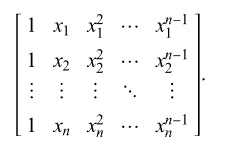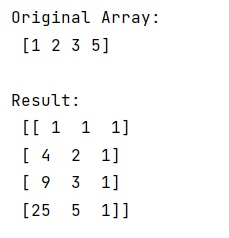# numpy.vander() Method with Example

Learn about the numpy.vander() method, how does it work?
Submitted by Pranit Sharma, on March 04, 2023

## Python - numpy.vander() Method

numpy.vander() method is used to generate a Vandermonde matrix. A Vandermonde matrix is a type of matrix that arises in the polynomial least squares fitting, Lagrange interpolating polynomials, and the reconstruction of a statistical distribution from the distribution's moments. A Vandermonde matrix of order is of the following form:The columns of the output matrix are powers of the input vector. The order of the powers is determined by the increasing boolean argument. Specifically, when increasing is False, the i-th output column is the input vector raised element-wise to the power of N - i - 1.

### Syntax

```numpy.vander(x, N=None, increasing=False)
```

### Parameter(s)

• x: input array (1D)
• n: int values which represent the number of columns in the output.
• increasing: Order of the powers of the columns.

Let us understand with the help of an example,

## Python program to demonstrate the example of numpy.vander() Method

```# Import numpy
import numpy as np

# Creating a numpy array
arr = np.array([1, 2, 3, 5])

# Display original array
print("Original Array:\n",arr,"\n")

# Creating a vandermonde matrix
res = np.vander(arr,3)

# Display result
print("Result:\n",res)
```

### OutputLanguages: » C » C++ » C++ STL » Java » Data Structure » C#.Net » Android » Kotlin » SQL
Web Technologies: » PHP » Python » JavaScript » CSS » Ajax » Node.js » Web programming/HTML
Solved programs: » C » C++ » DS » Java » C#
Aptitude que. & ans.: » C » C++ » Java » DBMS
Interview que. & ans.: » C » Embedded C » Java » SEO » HR
CS Subjects: » CS Basics » O.S. » Networks » DBMS » Embedded Systems » Cloud Computing
» Machine learning » CS Organizations » Linux » DOS
More: » Articles » Puzzles » News/Updates